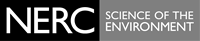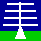The NERC MST Radar Facility at Aberystwyth

# Beam broadening of spectral widthSince the (two-way) polar diagram of a vertically directed radar beam has a Gaussian form: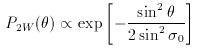where the standard deviation σ0 represents the beam-width, the spread of Doppler velocities, vD, as a result of beam-broadening will also have a Gaussian form centred about the mean vertical velocity: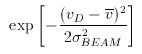where the standard deviation &sigmaBEAM is given by: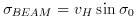The spread of velocities caused by turbulent activity will also have a Gaussian distribution about the mean vertical velocity, with a standard deviation σTURB which gives a measure of the turbulence intensity, and so the standard deviation of the combined distributions, i.e. the spectral width of the radar return signal, σSW, is given by: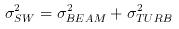Note that the spectral widths in the old format files of NERC MST Radar data are defined in a non-standard way; the values must therefore be multiplied by a scaling factor of 1.25 (explained in the file format page) before the following correction can be applied. The variance of vertical velocity variations caused by turbulence can therefore (in principle) be extracted from the observed spectral widths through the relationship:The diagram below shows a scatter plot of observed vertical beam spectral widths as a function of horizontal wind speed for 24 hour's worth of observations made by the NERC MST radar. The red line corresponds to vHsinσ0, i.e. σBEAM, where sin2σ0 = 2.47 × 10-4, which corresponds to a one-way half-power half-width of 1.5°. It should be noted that beam broadening corrected values of spectral can only be extracted for points lying above the line. Moreover, attention should confined to those points which lie significantly above the line. Since the beam broadening contribution to the observed spectral width increases with increasing wind speed, the liklihood of being able to extract turbulence information decreases with increasing wind speed.